# ISEE Upper Level Quantitative : Mode

## Example Questions

← Previous 1

### Example Question #1 : Mode

Examine the data set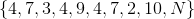. You are given thatcan be any integer from 1 to 10.

Which value(s) ofwould make 4 the only mode of the data set?

Only 4 or 7.

Any integer from 1 to 10.

Any integer from 1 to 10 except 7.

Only 4.

Any integer from 1 to 10 except 4 or 7.

Any integer from 1 to 10 except 7.

Explanation:

The mode of a data set is the element that appears most frequently.

The data set has ten elements - one 2, one 3, three 4's, two 7's, one 9, one 10, and an element of unknown value.

If that unknown element is 4, then there will be four 4's, and two or fewer of any other value, making 4 the only mode.

If that unknown element is 7, then there will be three 4's, three 7's, and one of each of the other elements, making 4 and 7 the modes.

If the unknown element is any other integer, then there will be three 4's and no more than two of any other element, making 4 the only mode.

The correct choice is therefore any integer other than 7.

### Example Question #2 : How To Find Mode

Examine the data set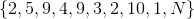. You are given thatcan be any integer from 1 to 10.

Which value(s) ofwould give the data set exactly one mode?

Any integer from 1 to 10 except 6, 7, or 8

Only 2 or 9

Any integer from 1 to 10 except 2 or 9

Any integer from 1 to 10

Only 6, 7, or 8

Only 2 or 9

Explanation:

The mode of a data set is the element that appears most frequently.

Two values of the data set, 2 and 9, are known to appear twice; the others appear exactly once.

Ifis 6, 7, or 8, this does not change, and 2 and 9 are both modes.

Ifis 1, 3, 4, 5, or 10, then three different values appear twice, and 2, 9, and the choice ofare the three modes.

Ifis 2 or 9, then that number appears three times, the other number appears twice, and all others appear once. That makes the choice ofthe only mode.

This makes the correct choice 2 or 9.

### Example Question #1 : Mode

Examine the data set. You are given thatcan be any integer from 1 to 10.

Which value(s) ofwould make 7 the only mode of the data set?

Only 7.

Only 4 or 7.

Any integer from 1 to 10.

This is impossible for any value of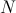.

Any integer from 1 to 10 except 4.

This is impossible for any value of.

Explanation:

The mode of a data set is the element that appears most frequently.

The data set has ten elements - one 2, one 3, three 4's, two 7's, one 9, one 10, and an element of unknown value.

If that unknown element is 7, there are two modes of the set, 4 and 7, which would occur three times each, as opposed to the other elements, which would appear once each.

If that unknown element is any other integer, then the only mode of the data set is 4, which would occur three times, as opposed to the other elements, which would appear once or twice each.

The correct choice is that this impossible.

### Example Question #4 : How To Find Mode

Consider the data set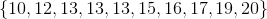Which is the greater quantity?

(a) The median of the data set

(b) The mode of the data set

(a) is greater

(b) is greater

It is impossible to tell from the information given

(a) and (b) are equal

(a) is greater

Explanation:

(a) The median of a data set with ten elements is the arithmetic mean of the fifth-highest and sixth-highest elements. These elements are 13 and 15, so the median is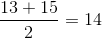(b) The mode of a data set is the element that occurs most frequently. Since 13 is the only repeated element, it is the mode.

This makes (a) the greater quantity.

### Example Question #5 : How To Find Mode

Consider the data set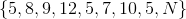,

whereis unknown.

Which is the greater quantity?

(a) The mode of the data set

(b) 5

(a) is greater.

(b) is greater.

(a) and (b) are equal.

It is impossible to tell from the information given.

(a) and (b) are equal.

Explanation:

5 appears in the data set at least three times. Regardless of the value of, no other element (except 5) can appear more than twice. 5 must be the mode.

### Example Question #6 : How To Find Mode

Consider the following set of data: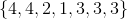compare the mode and the median of the set.

The median is greater.

It is not possible to compare based on the information given.

The mode is greater.

The median and the mode are equal.

The median and the mode are equal.

Explanation:

If there are an odd number of values in a data set, the median is the middle value. In this problem first we should put the numbers in order: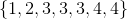So the median is.

The mode of a set of data is the value which occurs most frequently, which isin this problem, which has occured three times in the given set of numbers. So the median and the mode are equal.

### Example Question #7 : How To Find Mode

Compare the median and the mode in the following set of data: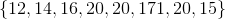The mode is greater than the median.

The mode and the median are equal.

It is not possible to copare the median and the mode based on the information given.

The median is greater than the mode.

The mode is greater than the median.

Explanation:

If a data set has an even number of values, the median is the average of the two middle values. First we should put the numbers in order: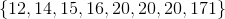So the median is: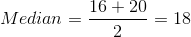The mode of a set of data is the value which occurs most frequently, which isin this problem. So the mode is greater than the median.

### Example Question #1 : How To Find Mode

Compare the median and the mode in the following set of data: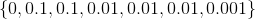The median and the mode are equal.

The median is greater than the mode.

It is not possible to compare the mode and the median based on the information given.

The mode is greater than the median.

The median and the mode are equal.

Explanation:

If there are an odd number of values in a data set, the median is the middle value. In this problem first we should put the numbers in order: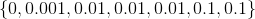So the median is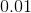.

The mode of a set of data is the value which occurs most frequently, which is. So the median and the mode are equal.

### Example Question #9 : How To Find Mode

Compare the mean and the mode in the following set of data: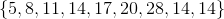It is not possible to compare the mean and the mode based on the information given.

The mean and the mode are equal.

The mean is greater than the mode.

The mode is greater than the mean.

The mean is greater than the mode.

Explanation:

The mode of a set of data is the value which occurs most frequently, which in this case is.

The mean of a set of data is given by the sum of the data, divided by the total number of values in the set. So we can write: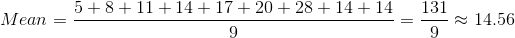So the mean of is greater than the mode.

### Example Question #10 : How To Find Mode

Compare the mean and the mode in the following set of data: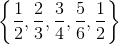The mode is greater than the mean.

The mean is greater than the mode.

It is not possible to compare the mean and the mode based on the information given.

The mean and the mode are equal.

The mean is greater than the mode.

Explanation:

The mode of a set of data is the value which occurs most frequently, which in this case is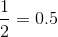.

The mean of a set of data is given by the sum of the data, divided by the total number of values in the set. So we can write: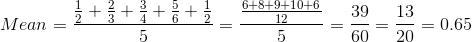So the mean is greater than the mode.

← Previous 1